# Using LET function in Excel with formula examples

This tutorial is introducing the new Excel LET function that makes intricate formulas look more comprehensible and calculate faster.

If you work with lengthy formulas in Excel, then you are certainly familiar with the idea of named ranges that make complex formulas easier to read. And now, Microsoft is making a step further and allows assigning names to calculations and values directly in a formula. If your formula uses the same expression several times, you can let Excel calculate it just once, store the result inside a formula and reuse as many times as needed. Sounds exciting, isn't it?

## Excel LET function

The Excel LET function allows you to assign names to calculation results and define variables inside a formula, so that the formula looks clearer and works faster.

Essentially, the concept is the same as naming cells, ranges and formulas in the Name Manager. What makes the LET function different is that the declared names only exist in the scope of a given formula and nowhere else.

## Syntax

LET has the following syntax and arguments:

LET (name1, name_value1, [name2], [name_value2], …, calculation)

Where:

• Name1 (required) - the first name to assign. It must begin with a letter.
• Name_value1 (required) - the value or calculation assigned to name1.
• Name2 / name_value2 (optional) - the second name and value.
• Calculation (required) - a calculation that uses assigned names and values.

The function can process up to 126 name/value pairs.

Note. Microsoft uses a slightly different syntax notation:

`LET(name1, name_value1, calculation_or_name2, [name_value2, calculation_or_name3…])`

I personally find it a little confusing, so I put it in a more customary form. Basically, they talk about the same thing but in different ways.

### Availability

The LET function is only available in Microsoft 365 subscriptions, including:

• Excel 365 for Windows
• Excel 365 for Mac
• Excel for the web

### Basic LET formula

If you do not have a clear understanding of the function yet, an example of the LET formula in its simplest form will help bring the essentials into focus.

Consider this simple expression: x+y. Here, we have 2 variables, x and y. Let's assign the value 2 to x, the value 5 to y and multiply one by the other:

`=LET(x, 2, y, 5, x*y)`

Enter this formula in a cell, and it will return 10 as the result.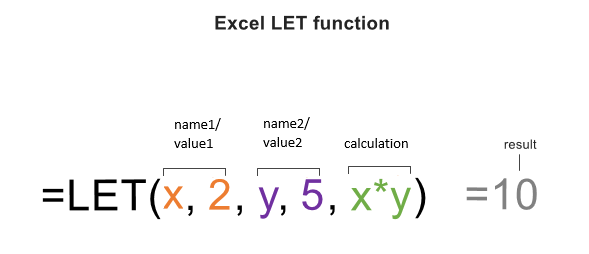Instead of values, the variables can be assigned to cell references, say x to B2 and y to B3:

`=LET(x,B2, y, B3, x * y)`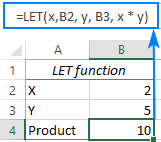To ease building a formula, the already declared names appear in the intellisense drop down list, just like the names defined in the name manager.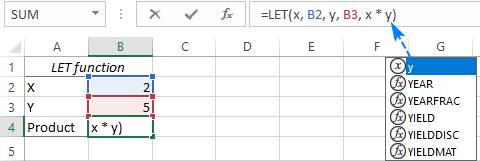Looking at the above formula you may be wondering, what's the point in over-complicating things? Why not simply put:

`=B2*B3`

In this particular case, a normal multiplication is of course simpler. The purpose is to clarify the concept. When it comes to more complex formulas with repeated calculations, the LET function takes on a whole new degree of usefulness as demonstrated in the further examples.

If you still doubt that LET will be a worthy addition to your Excel toolbox, then consider these benefits:

Simplified formulas. Giving descriptive names to variables and intermediate calculations makes it easier to understand what the formula is actually doing. You no longer need to figure out the inner logic of each specific expression or reference in a formula.

Faster calculations. When the same expression is repeated in a formula multiple times, Excel calculates it multiple times. When the expression is referred by name, Excel calculates it only once. As the result, a whole worksheet recalculates much faster. This positive effect on performance is especially noticeable in case of long formulas and huge data sets.

Easy maintenance. Using names for repeated calculations not only makes formulas more compact but also easier to update. When adjustments are needed, you make just a single change instead of updating the same expressions multiple times, thus saving time and reducing a change of human errors.

### Usage notes

To shorten the learning curve and prevent common errors, please follow these simple guidelines:

• A LET formula should have an odd number of arguments - one or several name/value pairs and a calculation. The last argument must always be a calculation that returns the final result.
• In your formulas, adhere to the same naming rules as in the name manager, i.e. start with a letter, no spaces, no punctuation characters, etc.
• To avoid confusion, avoid declaring names that are already used in the name manager. If the same name is assigned inside LET and defined in the name manager, the name manager version will be ignored.
• The names declared with LET are only valid in the scope of that particular formula.

## How to use LET function in Excel - formula examples

And now, it's time to look at more realistic use cases and reveal the whole power of the new function.

### Example 1. LET formula to reduce repeated calculations

When writing multi-level formulas, it often happens that the same expression or calculation is used more than once. A typical example is nested IF statements. In this context, let's see how LET can simplify things.

Supposing you have the results of student exams in three different subjects (columns B, C and D). You want to find an average for each student and grade it as shown in the table on the right: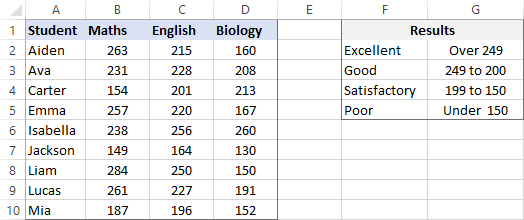The AVERAGE function can easily compute an arithmetic mean for each row:

`AVERAGE(B2:D2)`

And then, you build a nested IF statement based on the above criteria.

`=IF(AVERAGE(B2:D2)>249, "Excellent", IF(AVERAGE(B2:D2)>=200, "Good", IF(AVERAGE(B2:D2)>150, "Satisfactory", "Poor")))`

The problem is the same AVERAGE function is repeated three times. Well, maybe not really a problem, but an unnecessary complication that forces Excel to perform the same calculation thrice.

To optimize the formula, we can assign a name to the AVERAGE function (say, avg) and replace the function with this "local" name. This way, an average is calculated just once helping the formula run faster:

`=LET(avg, AVERAGE(B2:D2), IF(avg>249, "Excellent", IF(avg>=200, "Good", IF(avg>150, "Satisfactory", "Poor"))))`

Enter the formula in E2, drag it down through E10, and you'll get this result: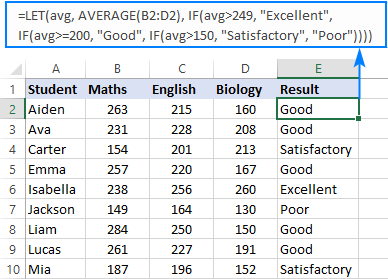Imagine you add one more exam (column E) to the source table. Naturally, you wish to include that in the average. Without LET, you'd have to perform this adjustment in the logical test of every IF function. Being a human being, you might overlook something and fail to update all the references correctly. With LET, you make just a single change:

`=LET(avg, AVERAGE(B2:E2), IF(avg>249, "Excellent", IF(avg>=200, "Good", IF(avg>150, "Satisfactory", "Poor"))))`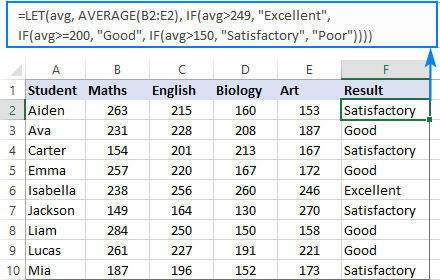### Example 2. LET with multiple names

Here's another example showing how the LET function can ease the creation of complex formulas.

Let's say you have a list of full names (column A) from which you wish to extract middle names. The below formula does the job perfectly, but at first sight its logic is quite obscure:

`=MID(A2, SEARCH(" ", A2) + 1, SEARCH(" ", A2, SEARCH(" ", A2) +1) - SEARCH(" ", A2) - 1)`

To provide some meaningful context to yourself and other users, you can define a couple of names such as:

• full_name - A2
• space - SEARCH(" ", full_name)

Obviously, cell A2 contains a full name that needs to be split, and the SEARCH function returns the position of the first space character in the name.

And then, we replace the cell reference and the SEARCH function with the declared names.

`=LET(full_name, A2, space, SEARCH(" ", full_name), MID(full_name, space + 1, SEARCH(" ", full_name, space + 1) - space - 1))`

If you are familiar with the MID syntax, the formula becomes much easier to comprehend:

To the position of the first space, you add 1 to start the extraction from the next character (space+1). To get the length of the middle name (i.e. how many characters to extract from the full name), you determine the position of the second space by nesting one SEARCH function into another, then find the difference between the positions of the two spaces and subtract 1 from the result to eliminate a trailing space (SEARCH(" ", full_name, space+1) - space -1)).

For better readability, you can warp the names and calculation onto separate lines like this: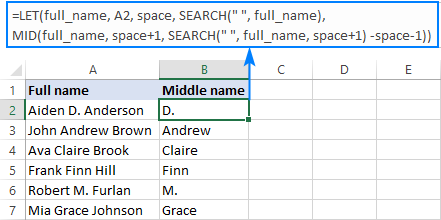### Example 3. LET function with dynamic arrays

In the previous examples, the LET function operated on a single cell, and we copied the formula to the below cells. However, LET can also accept arrays as input and produce arrays as output. This is possible due to Excel 365's new calculation engine and dynamic arrays.

From the below table, suppose you want to get a list of exams that are to be taken in the next n days, not including today. This can be done by using the FILTER function with multiple criteria:

`FILTER(data, (dates>today) * (dates<=today+n), "No results")`

All you need to do is to define the corresponding names:

• data - A2:C19
• dates - C2:C19
• today - TODAY()
• n - F3

After that, put the names and FILTER formula inside LET:

`=LET(data, A2:C19, dates, C2:C19, today, TODAY(), n, F3, FILTER(data, (dates>today) * (dates<=today+n), "No results"))`

The result is an array of records matching the specified criteria: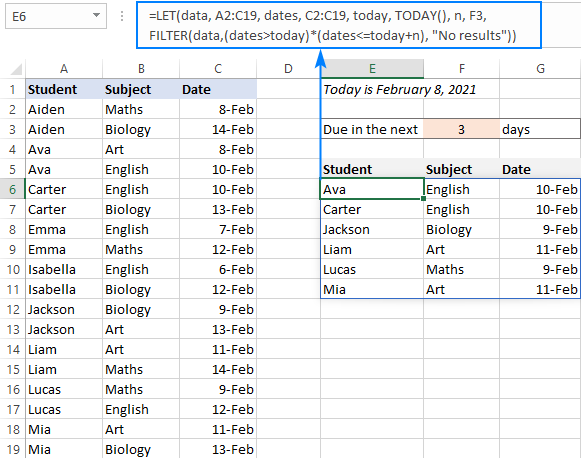## Excel LET function not working

If you cannot find the LET function in your Excel or your formula results in error, that may happen because of the following reasons:

### There is no LET function in my Excel

At the time of writing, the LET function is only available to Office Insiders. When the beta stage is completed, it will be released to all Microsoft 365 subscribers. The function is not backward compatible and won't appear in earlier Excel versions.

### #NAME? error

As there's a very slim chance that someone may misprint the function's name, the problem is most likely with assigned names. Please make sure you've declared each name used in the calculation and all of the names are spelled correctly.

That's how you can simplify and speed up your calculations with the help of the LET function. I thank you for reading and hope to see you on our blog next week!